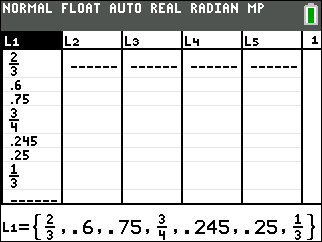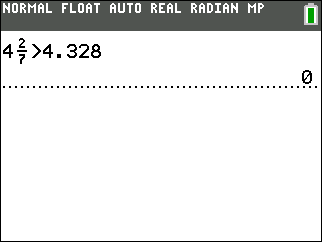••• ##### Device
• TI-83 Plus Family
• TI-84 Plus
• TI-84 Plus Silver Edition
•TI-84 Plus C Silver Edition
•TI-84 Plus CE

TI Connect™

# Middle Grades Math: Ordering Fractions, Decimals, and Percents

by Texas Instruments#### Overview

Students explore numbers and number representations for fractions, decimals, and percents.

#### Key Steps

•Students will order and compare numbers, including fractions, decimals, and percents. Students will use the Sort command on a given list to determine the greatest and least number.

They will then convert the list from fractions to decimals all at one time to determine if any of the numbers are equal.

•Finally, students use the Boolean operators, <, >, and =, to test if given statements are true or false. They will then place all of the numbers on a number line.